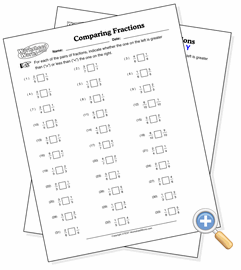# Comparing FractionsDetermine the value relationship between two fractions

This worksheet asks the student to identify which fraction in a pair is the larger by using the standard mathematical greater-than and less-than symbols. There are four levels of difficulty to select from. The lowest level produces pretty obvious problems, but the highest level problems may be challenging even to math teachers.